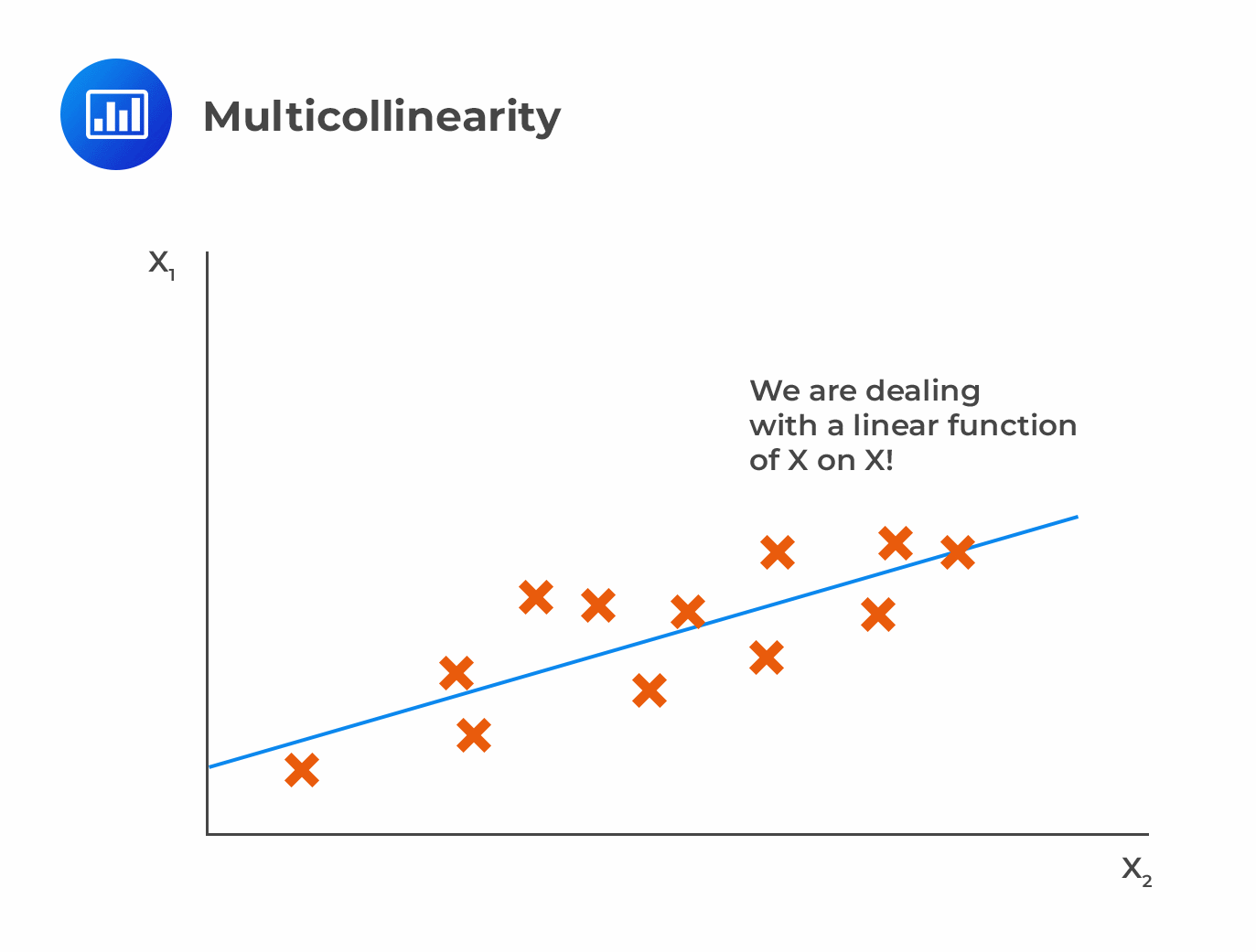# Multicollinearity

Multicollinearity occurs when two or more independent variables are significantly correlated to each other.It results from the violation of the multiple regression assumptions that there is no apparent linear relationship between two or more of the independent variables. Multicollinearity is common with financial data.

## Effects of Multicollinearity

Multicollinearity does not alter the consistency of the regression estimates. However, it renders them imprecise and unreliable. Multicollinearity makes it nearly impossible to determine how the independent variables influence the dependent variable individually. This inflates the standard errors for the regression coefficients, increasing the possibility of Type II errors.

## Detecting Multicollinearity

A high value of R2 and a significant F-statistic that contradicts the t-test signals multicollinearity. The insignificant t-statistic implies that the standard errors are overestimated. In addition, a high correlation between independent variables indicates multicollinearity. It is important to note that a low correlation between independent variables does not imply the absence of multicollinearity.

## Correcting Multicollinearity

The most common way to attenuate the problem of multicollinearity is to exclude some of the regression variables, provided that we do not omit relevant variables. However, it is hard to identify the variables that need to be eliminated. This is why stepwise regression is employed to systematically eliminate variables from the regression until multicollinearity is minimized.

Finally, we can summarize the problems in linear regression and their solutions as per the following table:

$$\small{\begin{array}{l|l|l}\textbf{Problem} & \textbf{Effect} & \textbf{Solution}\\ \hline\text{Heteroskedasticity} & \text{Incorrect standard errors} & \text{Use robust standard errors}\\ \hline\text{Serial correlation} & \text{Incorrect standard errors} & \text{Use robust standard errors}\\ \hline\text{Multicollinearity} & \text{High R-squared and low t-statistics} & \text{Eliminate one or more independent variables}\\ \hline{}& {}& \text{Stepwise regression}\\ \end{array}}$$

## Question

The regression problem that will most likely increase the chances of making Type II errors is:

A. Multicollinearity.

B. Conditional heteroskedasticity.

C. Positive serial correlation.

### Solution

Multicollinearity makes the standard errors of the slope coefficients to be artificially inflated. This increases the likelihood of incorrectly concluding that a variable is not statistically significant (Type II error).

B is incorrect. Conditional heteroskedasticity underestimates standard errors while the coefficient estimates remain unaffected. This inflates the t-statistics leading to the frequent rejection of the null hypothesis of no statistical significance. (Type I error).

C is incorrect. Positive serial correlation makes the ordinary least squares standard errors for the regression coefficients to underestimate the true standard errors. This inflates the estimated t-statistics, making them appear to be more significant than they really are. This increases Type I error.

LOS 2 (l) Describe multicollinearity and explain its causes and effects in regression analysis.

Shop CFA® Exam Prep

Offered by AnalystPrepLevel I
Level II
Level III
All Three Levels
Featured Shop FRM® Exam PrepFRM Part I
FRM Part II
FRM Part I & Part II
Learn with Us

Subscribe to our newsletter and keep up with the latest and greatest tips for success
Shop Actuarial Exams PrepExam P (Probability)
Exam FM (Financial Mathematics)
Exams P & FMGMAT® Complete CourseDaniel Glyn
2021-03-24
I have finished my FRM1 thanks to AnalystPrep. And now using AnalystPrep for my FRM2 preparation. Professor Forjan is brilliant. He gives such good explanations and analogies. And more than anything makes learning fun. A big thank you to Analystprep and Professor Forjan. 5 stars all the way!michael walshe
2021-03-18
Professor James' videos are excellent for understanding the underlying theories behind financial engineering / financial analysis. The AnalystPrep videos were better than any of the others that I searched through on YouTube for providing a clear explanation of some concepts, such as Portfolio theory, CAPM, and Arbitrage Pricing theory. Watching these cleared up many of the unclarities I had in my head. Highly recommended.Nyka Smith
2021-02-18
Every concept is very well explained by Nilay Arun. kudos to you man!2021-02-13